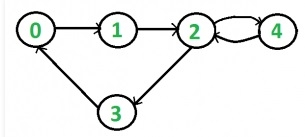# C++ Program to Check the Connectivity of Directed Graph Using BFS

To check connectivity of a graph, we will try to traverse all nodes using any traversal algorithm. After completing the traversal, if there is any node, which is not visited, then the graph is not connected.For the directed graph, we will start traversing from all nodes to check connectivity. Sometimes one edge can have the only outward edge but no inward edge, so that node will be unvisited from any other starting node.

In this case the traversal algorithm is recursive BFS traversal.

Input − Adjacency matrix of a graph

 0 1 0 0 0 0 0 1 0 0 0 0 0 1 1 1 0 0 0 0 0 1 0 0 0

Output − The Graph is connected.

## Algorithm

### traverse(s, visited)

Input: The start node s and the visited node to mark which node is visited.

Output: Traverse all connected vertices.

Begin
mark s as visited
insert s into a queue Q
until the Q is not empty, do
u = node that is taken out from the queue
for each node v of the graph, do
if the u and v are connected, then
if u is not visited, then
mark u as visited
insert u into the queue Q.
done
done
End

### isConnected(graph)

Input − The graph.

Output − True if the graph is connected.

Begin
define visited array
for all vertices u in the graph, do
make all nodes unvisited
traverse(u, visited)
if any unvisited node is still remaining, then
return false
done
return true
End

## Example Code

Live Demo

#include<iostream>
#include<queue>
#define NODE 5
using namespace std;
int graph[NODE][NODE] = {
{0, 1, 0, 0, 0},
{0, 0, 1, 0, 0},
{0, 0, 0, 1, 1},
{1, 0, 0, 0, 0},
{0, 1, 0, 0, 0}};
void traverse(int s, bool visited[]) {
visited[s] = true; //mark v as visited
queue<int> que;
que.push(s);//insert s into queue
while(!que.empty()) {
int u = que.front(); //delete from queue and print
que.pop();
for(int i = 0; i < NODE; i++) {
if(graph[i][u]) {
//when the node is non-visited
if(!visited[i]) {
visited[i] = true;
que.push(i);
}
}
}
}
}
bool isConnected() {
bool *vis = new bool[NODE];
//for all vertex u as start point, check whether all nodes are visible or not
for(int u; u < NODE; u++) {
for(int i = 0; i < NODE; i++)
vis[i] = false; //initialize as no node is visited
traverse(u, vis);
for(int i = 0; i < NODE; i++) {
if(!vis[i]) //if there is a node, not visited by traversal, graph is not connected
return false;
}
}
return true;
}
int main() {
if(isConnected())
cout << "The Graph is connected.";
else
cout << "The Graph is not connected.";
}

## Output

The Graph is connected.